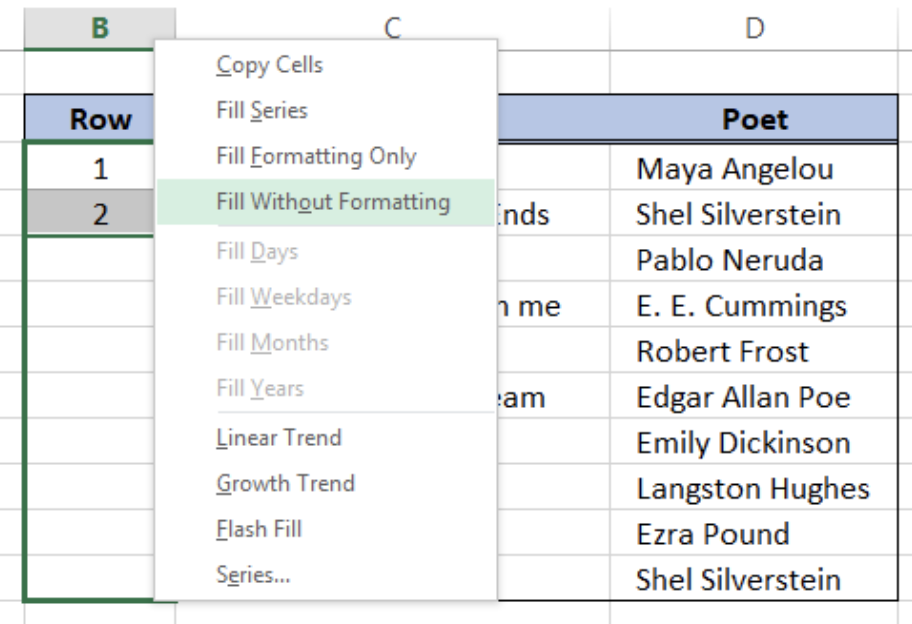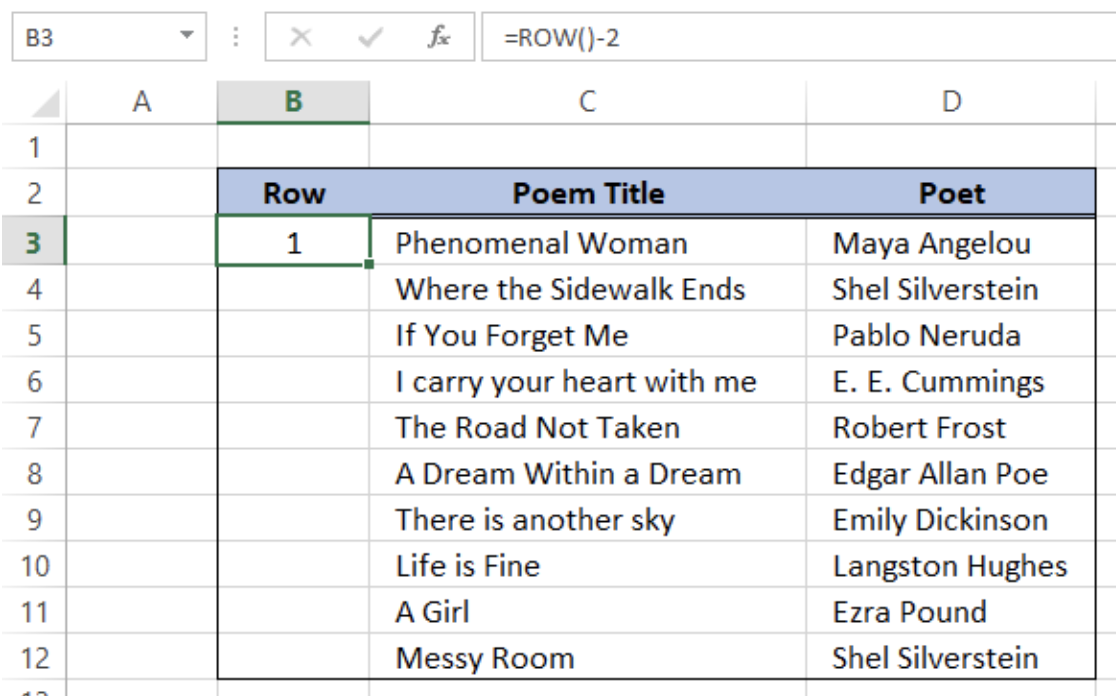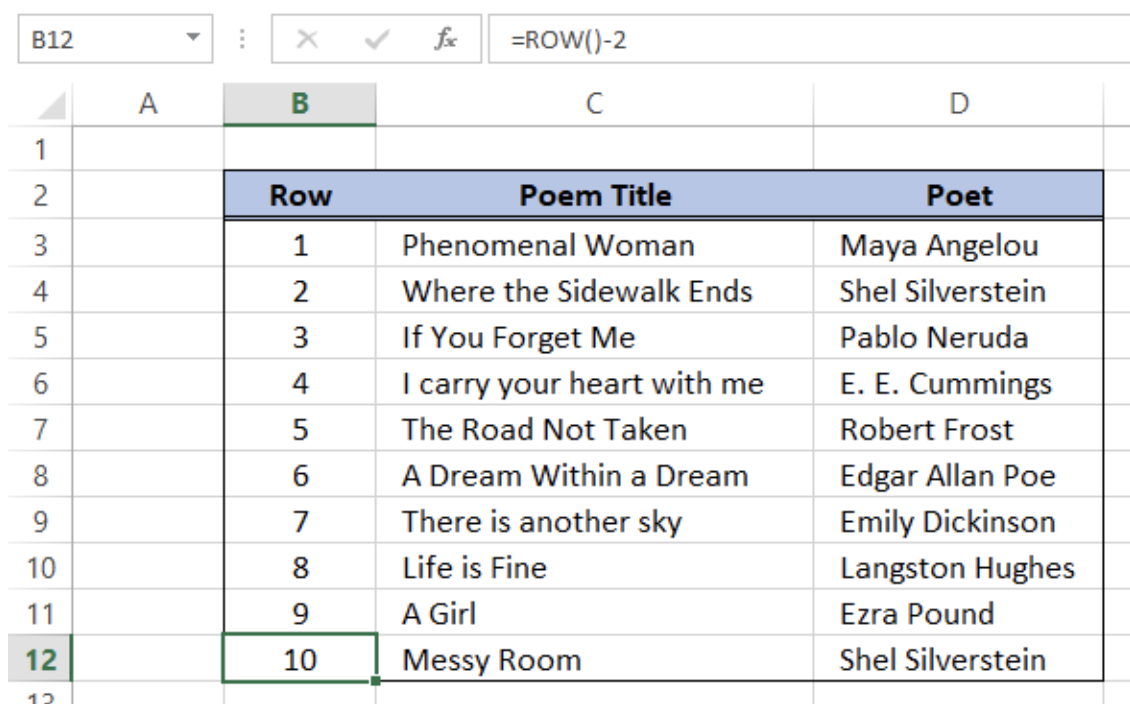Get instant live expert help with Excel or Google Sheets“My Excelchat expert helped me in less than 20 minutes, saving me what would have been 5 hours of work!”

#### Post your problem and you’ll get expert help in seconds.

Your message must be at least 40 characters
Our professional experts are available now. Your privacy is guaranteed.

# How to number cells

We can number cells in Excel by using the fill handle, or we can automatically number rows by using the ROW function.  This article assists all levels of Excel users on how to number cells.Figure 1.  Final result: How to number cells

## Numbering rows

• Select the cell where we want to insert the first row number, say B3, and enter “1”Figure 2.  Entering the first row number

• Type another value in the next cell to establish a pattern.  Enter “2” in cell B4.Figure 3.  Entering the second row number

• Select cells B3 and B4.  The fill handle will appear at the bottom right corner of the selected cells.Figure 4.  Selecting the first two cells to establish pattern

• Click, hold and drag the fill handle down until the last cell we want to fill.Figure 5.  Output: Numbering rows

We have now filled the column for row with numbers 1 to 10.  However, we have also copied the formatting of cells B3 and B4 and it has distorted the format of our table.

## Fill without formatting

In order to fill the row numbers without formatting, we have to drag down the fill handle using the riight-click button of the mouse.  When we release the mouse, options will be provided and we can choose Fill without Formatting to achieve the desired result.Figure 6.  Fill without Formatting option

Finally, we have filled all rows with a row number without distorting the current format of cells.Figure 7.  Output: Numbering without formatting

## Auto numbering

Next, let us learn how to automatically number rows by using the ROW function and an offset value.

• Select the first cell where we want to insert the first row number, say B3
• Enter the sequence formula using the ROW function

`= ROW()-2`Figure 8.  Entering the sequence formula

The ROW function returns the row number of the cell in the worksheet.  We have selected cell B3 so the ROW function returns the value 3. Therefore, we need to subtract 2 from the ROW function in order to obtain row number “1” for the first row.

The formula for sequential numbering is therefore given as:

`=ROW()-[offset]`

In this case, the offset is the number 2.

• Click the fill handle of cell B3 using the right-click button, and drag down to cell B12.
• Choose Fill without Formatting to copy only the formula.Figure 9.  Output: Auto numbering using ROW function

Note that the formula in cell B12 is the same as the formula in cell B3.  Finally, we have learned how to number cells by using sequential row numbers.

## Instant Connection to an Excel Expert

Most of the time, the problem you will need to solve will be more complex than a simple application of a formula or function. If you want to save hours of research and frustration, try our live Excelchat service! Our Excel Experts are available 24/7 to answer any Excel question you may have. We guarantee a connection within 30 seconds and a customized solution within 20 minutes.